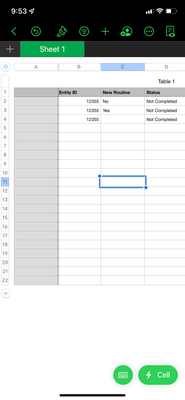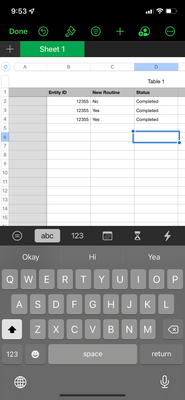cancel
Showing results for
Did you mean:Regular Visitor

## Distinct Count with Multiple Conditions

How can I create a distinct count with this condition? Status column will be completed once the new routine column has value.1 ACCEPTED SOLUTIONSuper User

``````Status =
VAR T1 =
CALCULATETABLE ( 'Table 1', ALLEXCEPT ( 'Table 1', 'Table 1'[Entity ID] ) )
VAR T2 =
FILTER ( T1, 'Table 1'[New Routine] <> BLANK () )
RETURN
IF (
COUNTROWS ( T1 ) = COUNTROWS ( T2 )
&& COUNTROWS ( T2 ) > 1,
"Completed",
"Not Completed"
)``````
7 REPLIES 7Super User

``````Status =
VAR T1 =
CALCULATETABLE ( 'Table 1', ALLEXCEPT ( 'Table 1', 'Table 1'[Entity ID] ) )
VAR T2 =
FILTER ( T1, 'Table 1'[New Routine] <> BLANK () )
RETURN
IF (
COUNTROWS ( T1 ) = COUNTROWS ( T2 )
&& COUNTROWS ( T2 ) > 1,
"Completed",
"Not Completed"
)``````Regular Visitor

Would you be able to help me on this? For this entity #, if 1 is yes, then the result is satisfied.Regular VisitorSuper User
``````Status 2 =
VAR T1 =
CALCULATETABLE (
VALUES ( 'Table 1'[New Routine] ),
ALLEXCEPT ( 'Table 1', 'Table 1'[Entity ID] )
)
RETURN
IF ( "Yes" IN T1, "Satisfied", "Not Satisfied" )``````Regular Visitor

Did not workRegular Visitor

It works! Thank you!Regular Visitor

Up